Quotient

Determine the quotient (q) and the remainder (r) from division numbers 100 and 8. Take the test of correctness.

Result

q =  12
r =  4

Solution:Leave us a comment of example and its solution (i.e. if it is still somewhat unclear...):Be the first to comment!Next similar examples:

1. Like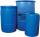When is in 14 barrels 140 liters of water, how many liters barrels of 8 liters I need to get all the water from the larger barrels?
2. DivisionWhich number in division 16 give 12 and the rest 3?
3. School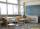27 students of 6.D class is going to a trip. They pay 9 € each. The teacher got 153 €. How many children have not paid?
4. The resultHow many times I decrease the number 1632 to get the result 24?
5. Crates 2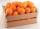One crate will hold 50 oranges. If Bob needs to ship 932 oranges, how many crates will he need?
6. DoctorsIn the city operates 171 doctors. The city has 128934 citizens. How many citizens are per one doctor?
7. Math classificationIn 3A class are 27 students. One-third got a B in math and the rest got A. How many students received a B in math?
8. Wallpaper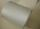Salesman account for 2.3 meters of wallpaper 149.50 Kc. How much cost 1 meter of wallpaper?
9. ChocolateChildren break chocolate first to third and then every part of another half. What kind got each child? Draw a picture. What part would have received if each piece have halved?
10. Valid numberRound the 453874528 on 2 significant numbers.
11. Write decimalsWrite in the decimal system the short and advanced form of these numbers: a) four thousand seventy-nine b) five hundred and one thousand six hundred and ten c) nine million twenty-six
12. ProductResult of the product of the numbers 1, 2, 3, 1, 2, 0 is:
13. To thousands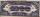Round to thousands following numbers:
14. Roman numeralsWrite numbers written in Roman numerals as decimal.Added together and write as decimal number: LXVII + MLXIVMy number's tens is three times more then ones My number's ones is twice the number of thousands and my number's hundreds is half the number of of tens. I have two ones. which number am I?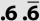Double round number 727, first to tens, then to hundreds. (double rounding)